### Day 09 - Limits Problems - 08.28.14

 Bell Ringer Tutoring OpportunityCreate a problem involving one of the concepts covered in chapter 1. Include an answer key with multiple ways to solve the problem. Evaluating Limits Algebraically Evaluating Limits Graphically Evaluating Limits Numerically Removable Discontinuity Nonremovable Discontinuity One-sided Limits Infinite Limits Stump Your Teacher Problems Review Finding limits using a table Finding limits using a graph Epsilon-Delta Limit Proofs Limit Properties Dividing Out/Rationalizing Techniques Functions that agree at all but one point One-sided Limits Infinite Limits Lesson Find the values of the constants a and b such that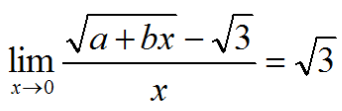Consider the function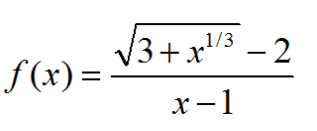Find the domain of f. Calculate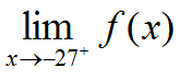Calculate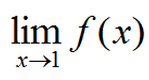Determine all value of the constant a such that the following function is continuous for all real numbers.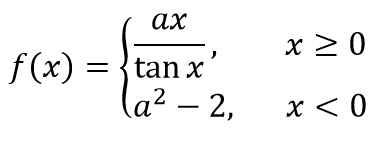Exit Ticket The function f and its graph are shown below: Calculate the limit of f(x) as x gets closer to 2 from the left side. Which value is greater? the limit of f(x) as x goes to 1 f(1) Explain your answer. At what value(s) of c on the interval [0, 4] does the limit of f(x) as x goes to c not exist? Explain your answer.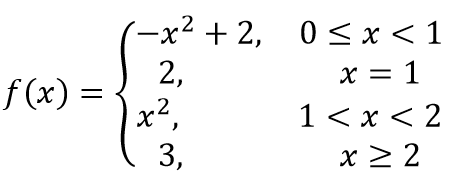Lesson Objective(s) How are the concepts in chapter 1 related? Standard(s) APC.2 Define and apply the properties of limits of functions. Limits will be evaluated graphically and algebraically. Includes: ​limits of a constant ​limits of a sum, product, and quotient ​one-sided limits ​limits at infinity, infinite limits, and non-existent limits*APC.3Use limits to define continuity and determine where a function is continuous or discontinuous.Includes:​continuity in terms of limitscontinuity at a point and over a closed interval​application of the Intermediate Value Theorem and the Extreme Value Theorem​geometric understanding and interpretation of continuity and discontinuityAPC.4Investigate asymptotic and unbounded behavior in functions.Includes:describing and understanding asymptotes in terms of graphical behavior and limits involving infinity